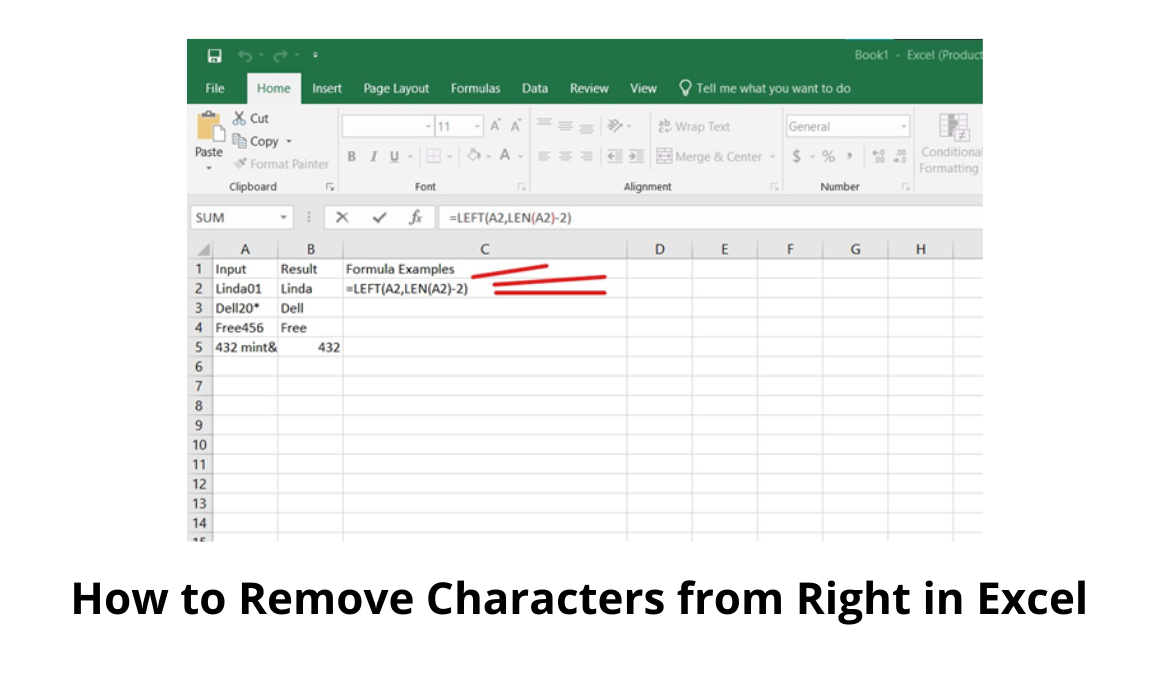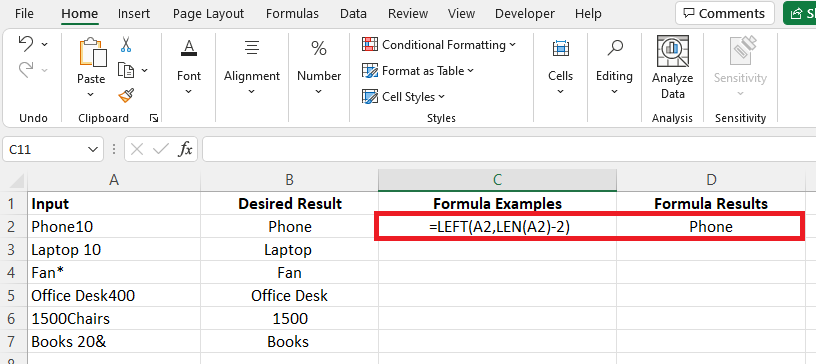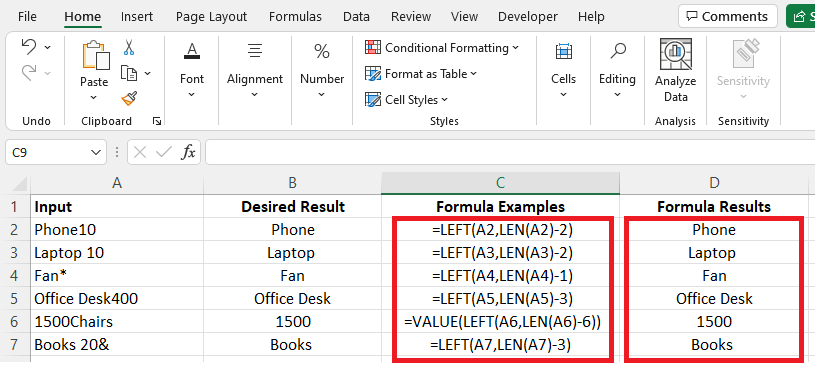# How to Remove Characters from Right in ExcelAlongside the many functions available for use in excel for efficient data organization and representation is the ability to remove characters or delete some part of a text string.

In this article, we will be showing you how to apply formulas and function syntaxes to remove characters from the right side of a text string.

Before we go on, let’s review the advantages of knowing how to remove characters from the right side of a text string:

• Knowing how to remove a character from the right side of a text string helps keep your data more organized.
• In case of an error in the input of data, we can easily harness this function to change our data, especially when we have a long list of data to work with.
• Data organization had never been more effortless until excel came, and so it’s profitable to know the various functions on excel that can make for a stress-free data representation and analysis.
• In the real world, time is a factor of productivity, so knowing how to remove characters from the right side of a text string will save you a lot of time and effort.
• Another excellent advantage of it is that the possibilities of making mistakes will be reduced in the computation of your dataset. When you begin to make changes to your dataset manually, especially when working with a large data bank, making mistakes and introducing errors into your computation is inevitable.

Now that I have shown you some of the very practical reasons for harnessing this function and applying it to your dataset, let’s now proceed to review the steps to make these changes.

## Here’s How to Remove Characters from Right in Excel

### Using the Generic Formula

You can use the generic formula for removing characters from the right side of a text in Excel.

In using the generic formula, however, you need to know that it involves two primary functions that you will be using.

The LEFT and LEN function.

Both functions have specific arguments that you must know and understand to be able to use them for making changes to your dataset.

LEFT function

• Text: the particular text string from which you want to extract
• The number of characters: the number of characters from the text string above that you intend to delete.

LEN function

Text: the text in a cell.

In order for the generic formulas to work correctly, we need to keep in mind the full length of the text string and the number of characters to be removed from it.

`=LEFT(text,LEN(text)-n)`

Let’s consider an example and then apply these formulas.

See the illustration below:The first thing to consider in the example above is that we count the number of characters we want to remove from the whole text string.

Let’s take that number to be “n”, then we apply our formula as used above.

`=LEN(A2)-2 = 5`

By doing this now, we know the length of the whole string and now we combine both LEFT and LEN functions to get the desired result.

`=LEFT(A2,LEN(A2)-2)`

`=LEFT(A2,5) = Phone`

Let me give further explanations on the use of LEFT and LEN functions

The LEFT method is ideal for pulling characters from the text string’s left side.

To extract all characters up to the number of characters we want to reduce, we utilize LEFT in this formula.

The problem with extracting characters from data of variable length is not knowing how many to extract.

The LEN function comes into play here.

The LEN function estimates the whole length span of values from the inside out.

The whole length of A6 (1500Chairs) is 10.

We deduct 6 from the total count of characters we wish to remove, which is the length of “1500” including the space character.

The result is 4, which is used by LEFT to determine how many characters to remove.

The text “1500” is returned as a text value by the LEFT function.

`=LEFT(A6,LEN(A6)-6)`

`=LEFT(A6,6) = 1500`

Apart from the LEFT and LEN functions, we might also need to use the VALUE function.

From the illustration above, we can see that in cell A6, we have 1500Chairs and on trying to remove “Chairs” we would have “1500” which is in text format, and because we want it to be numeric and not in text format, we then use the VALUE function to convert it to a numeric value in excel.

In integrating the VALUE function into your formula, you need to follow the below steps:

`=VALUE(LEFT(A6,LEN(A6)-6))`

`= VALUE(LEFT(A5,'10-6'))`

`= VALUE (LEFT (A5,4))`

`= VALUE (“1500”)`

`= 1500`

Check out the illustration below for a better understanding.Applying the formula for all the entries on the spreadsheet, we achieve the desired result in the “Formula Results”.## Conclusion

In this article, we’ve reviewed how to go about removing characters from already imputed data from the right side, and also we have identified the various functions that we need such as the LEFT, LEN, and VALUE functions.

Accurately following the steps in this tutorial will make getting the desired results much easier.

Although Excel is not case sensitive, when it comes to executing commands, it will only work out a command when the right symbols.

Be sure to pay attention to the symbols that you input into the command strings.

For example, the correct bracket to use is () and not [] or {}.

I hope this piece was worth your time and more!

Ovo is a highly specialized Computer and Networking Expert with experience in Windows, Cisco, Microsoft Office (Excel, Word, etc) and Networking engineering. Ovo is a creative, team player that loves sharing his experience with technology with readers to follow along. He has great attention to detail when discussing various technologies, tutorials and guides.